Would you like to react to this message? Create an account in a few clicks or log in to continue.

# Time as a Variable in the Function Element

2 posters

#### KerimF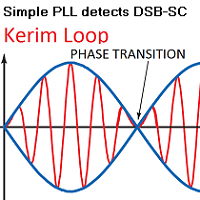Since analog voltages can be used as variables in the formula of a function element, I wonder if ‘time’ could also be made to be used as a variable.

#### KerimFA workaround is to use 1A source supplying a 1F capacitor.

The capacitor voltage will equal the charging time (assuming Vc=0 at the start) which is equivalent to the simulator running time.

#### KerimFWhat could be wrong in the following formula (defined in a function element)?

vo0 = (vi0<0.500)*0 + (vi0>=0.500)*(vo0+vi1)/(vi0-0.480)

It gives ‘infinity’ for vi0>=0.500.
The input voltage ‘vi0’ equals ‘time’ of simulation.

Thank you.

#### arcachofoWhich simulide version?

EDIT:
In development version this case does not work.
In 1.0.1 seems to work.

#### KerimFarcachofo wrote:Which simulide version?

EDIT:
In development version this case does not work.
In 1.0.1 seems to work.

SimulIDE_1.0.1-R1386_Win32
By the way, this function element is included on a rather big circuit. Speed is around 000.26 % and Load is around 300 %

Odd Topic:
I noticed that the current value of the voltage source is not saved with the schematic.

#### KerimFSimulIDE_1.0.1-R1386_Win32

Please check the 'vo0=infinity' after 500ms in the following circuit (XF_3-2_08.sim1).Attachments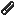XF_3-2_08.zip

#### arcachofoI didn't realyze before:
The correct notation is vo = ... not vo0 = ...

And this is not relevant but (vi0<0.500)*0 is always 0.

#### KerimFarcachofo wrote:I didn't realyze before:
The correct notation is vo = ... not vo0 = ...

And this is not relevant but (vi0<0.500)*0 is always 0.

I wrote 'vo0' instead of 'vo' because this is what is written on the symbol. I thought 'vo' was just a mistyping in the help page because the function element can have many inputs 'vi0', vi1', vi2'.... and many outputs as well 'vo0', 'vo1', vo2'... etc.

Doesn't this mean that also in the case of 1 input, the correct notation should be 'vi', not 'vi0' (as it is written on the symbol)?

While I am writing here, I re-tested the circuit after correcting the formula... the result, I got, is also a big number 'nan pV'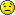#### arcachofoI wrote 'vo0' instead of 'vo' because this is what is written on the symbol. I thought 'vo' was just a mistyping in the help page because the function element can have many inputs 'vi0', vi1', vi2'.... and many outputs as well 'vo0', 'vo1', vo2'... etc.

Doesn't this mean that also in the case of 1 input, the correct notation should be 'vi', not 'vi0' (as it is written on the symbol)?
Each function is for one output, so there is only one output for each function.
You define the voltage for that output so it does not use any number, it is just the output voltage.
As values you can use any input or output voltages and you need to specify the number.

But I didn't remember that for version 1.0.1 anything starting by "vo" will work, even voZZ vo9837 or whatever.

The infinity is the result of the function:
Note that you are doing: vo0 = vo0+something
So it will grow and grow until it overflows.

And it does it very fast because vi0 = 0.5 then vi0-0.480 = 0.02 and vi1 = 96.8:
so the result is 4840 and then...
246828
1.2345e+07
6.17193e+08
3.0855e+10
1.54252e+12
7.71106e+13
3.85476e+15
1.9269e+17
...
inf

#### KerimFarcachofo wrote:
I wrote 'vo0' instead of 'vo' because this is what is written on the symbol. I thought 'vo' was just a mistyping in the help page because the function element can have many inputs 'vi0', vi1', vi2'.... and many outputs as well 'vo0', 'vo1', vo2'... etc.

Doesn't this mean that also in the case of 1 input, the correct notation should be 'vi', not 'vi0' (as it is written on the symbol)?
Each function is for one output, so there is only one output for each function.
You define the voltage for that output so it does not use any number, it is just the output voltage.
As values you can use any input or output voltages and you need to specify the number.

SimulIDE_1.0.1-R1386_Win32

It seems 'vo0' is equivalent to 'vo', in the case the element has 1 output.
How did I discover this?by just inspecting the ANSI lines of the sim1.
In the circuit, there are 3 function elements. The first two (having each 1 input, 1 output) accepted 'vo0' and worked well. And the third one (of interest) also accepted 'vo'.

The 'infinity' issue is indeed, as you described, due to using a wrong logic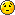which I have to solve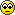. The object is to let 'vo' give the average of the charging current 'vi1'.

#### arcachofoOdd Topic:
I noticed that the current value of the voltage source is not saved with the schematic.
You are right, it happens in some cases.
Solved at trunk Rev 1428.

The state of the button is now also saved at trunk Rev 1427.